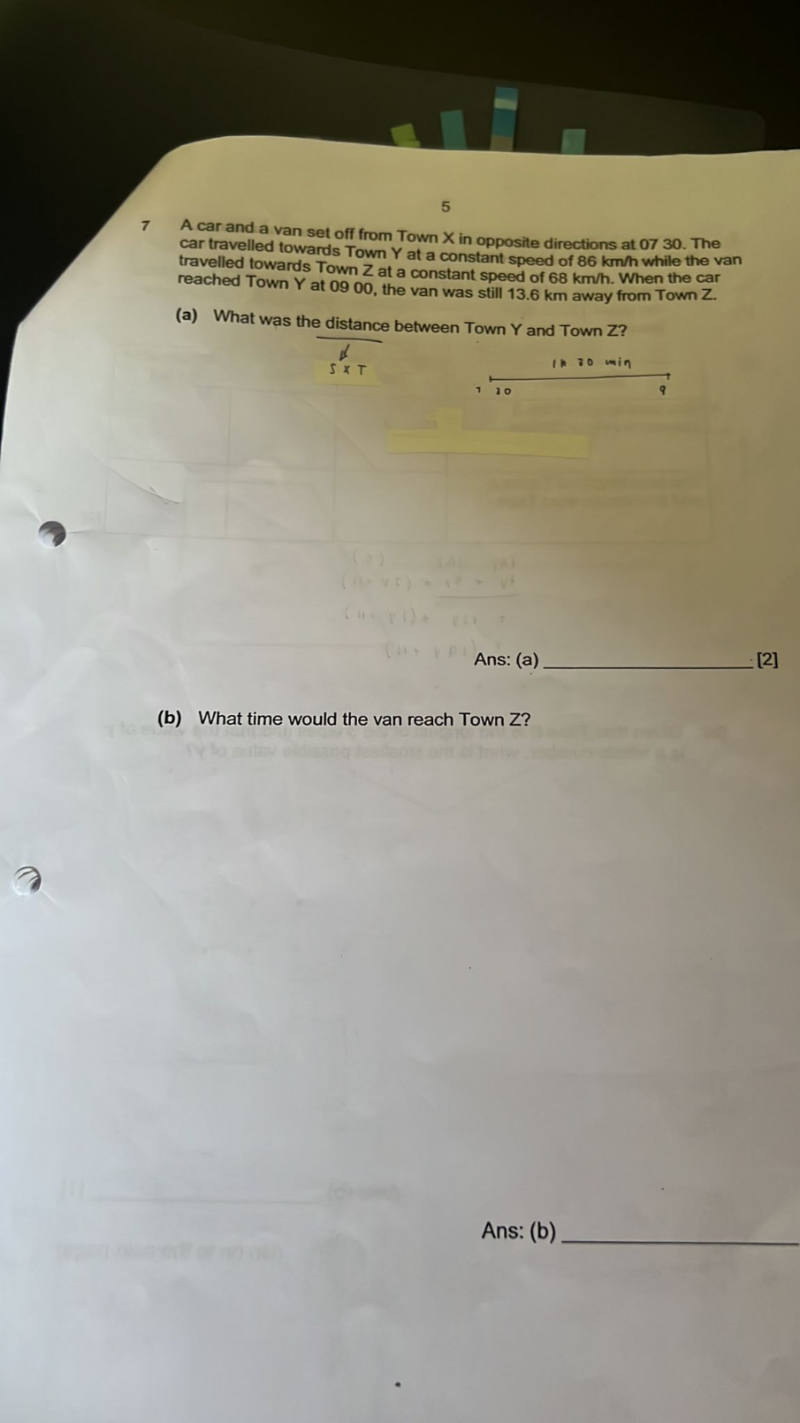# QuestionThank you

?                  9am               7:30am                                                   9am

Z <————|————–X ————————————->  Y

<-13.6–><—- d2——-><————— d1 ——————>

<—-  Van (678km/h) Car(86km/h)——->

(a)   Car speed = 86km/h,      Time taken = 9.5-7 = 1.5h,    Distance d1 = 86 x 1.5 = 129km

Van speed = 68km/h       Time taken = 1.5                     Distance d2 = 68 x 1.5 = 102km

Therefore, distance between Y and Z = 13.6 + 102 + 129 = 244.6km

(b)  Remaining distance = 13.6

Time taken for remaining distance =  (13/6) / 68 = 0.2 h = 12 mins

|—–12m—-|

9am             9:12am

Hence the van reached Town Z at 9:12am

0 Replies 2 Likes ✔Accepted Answer